A neutron of mass m enters a uniform magnetic field B with the velocity v perpendicular to it. The radius of the circular path made by a neutron is :

1.

2.

3.

4.  $\mathrm{Zero}$

Concept Questions :-

Lorentz force
NEET 2021 - Achiever Batch - Aryan Raj Singh

Difficulty Level:

Two similar coils of radius R are lying concentrically with their planes at right angles to each other. The currents flowing in them are 3I and 4I respectively. The resultant magnetic field induction at the center is :

1.

2.

3.

4.   $\mathrm{Zero}$

Concept Questions :-

Magnetic field due to various cases
NEET 2021 - Achiever Batch - Aryan Raj Singh

Difficulty Level:

A current-carrying closed Loop in the form of a right isosceles triangle ABC is placed in xy plane in a uniform magnetic field acting along the y-axis as shown in the figure. If the magnetic force on the arm AC is $\stackrel{\to }{F}$, then force on the arm BC and AB are respectively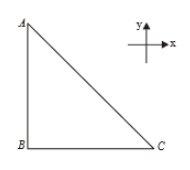(1) zero, zero

(2)

(3)

(4)

Concept Questions :-

Lorentz force
NEET 2021 - Achiever Batch - Aryan Raj Singh

Difficulty Level:

A uniform electric field and a uniform magnetic field are acting in the same direction in a certain region. If a proton is projected in this region such that its velocity is pointed along the direction of fields, then the proton :

1.  will turn towards the left of the direction of motion

2.  will turn towards the right of the direction of motion

3.  will speed up

4.  will slow down

Concept Questions :-

Lorentz force
NEET 2021 - Achiever Batch - Aryan Raj Singh

Difficulty Level:

When a proton is released from rest in a room, it starts with an initial acceleration ${\mathrm{a}}_{0}$ towards the east. When it is projected towards the north with a speed ${\mathrm{v}}_{0}$, it moves with initial acceleration $3{\mathrm{a}}_{0}$ towards east. The electric and magnetic fields in the room are -

1.

2.

3.

4.

Concept Questions :-

Lorentz force
NEET 2021 - Achiever Batch - Aryan Raj Singh

Difficulty Level:

1.  In a circle

2.  In y-z plane

3.  With constant speed

4.  All of these are correct

Concept Questions :-

Lorentz force
NEET 2021 - Achiever Batch - Aryan Raj Singh

Difficulty Level:

If the planes of two identical concentric coils are the perpendicular and magnetic moment of each coil is M, then the resultant magnetic moment of the two coil system is

1.  M

2.  $\sqrt{2}\mathrm{M}$

3.  3M

4.  2M

Concept Questions :-

Magnetic moment
NEET 2021 - Achiever Batch - Aryan Raj Singh

Difficulty Level:

A current wire is hidden in a wall. Its position can be located with the help of a

1.  Moving coil galvanometer

2.  Voltmeter

3.  Hotwire ammeter

4.  Magnetic needle

Concept Questions :-

Magnetic field due to various cases
NEET 2021 - Achiever Batch - Aryan Raj Singh

Difficulty Level:

Which of the following graph correctly represents the variation of magnetic field induction with distance due to a thin wire carrying current?

1.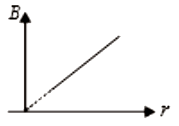2.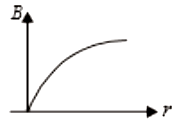3.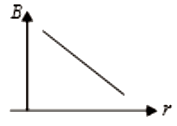4.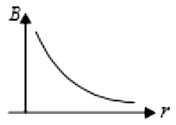Concept Questions :-

Magnetic field due to various cases
NEET 2021 - Achiever Batch - Aryan Raj Singh

Difficulty Level:

When an electron enters perpendicularly in a magnetic field with velocity v, time period of its revolution is T. If it enters in the same magnetic field with a velocity 2v, then its time period will be:

1.  2T

2.  4T

3.  $\frac{\mathrm{T}}{2}$

4.   T

Concept Questions :-

Lorentz force